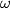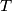### IMO Shortlist 2018 problem G3

Kvaliteta:
Avg: 0,0
Težina:
Avg: 7,0

A circle$\omega$ with radius$1$ is given. A collection$T$ of triangles is called good, if the following conditions hold:

(i) each triangle from$T$ is inscribed in$\omega$;

(ii) no two triangles from$T$ have a common interior point.

Determine all positive real numbers$t$ such that, for each positive integer$n$, there exists a good collection of$n$ triangles, each of perimeter greater than$t$.

Izvor: https://www.imo-official.org/problems/IMO2018SL.pdf